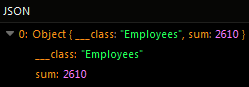# Retrieving Unique Objects¶

Unique objects can be retrieved from a column of the requested table by setting the `true` value in the `setDistinct` method, The `distinct` parameter is a boolean value which defaults to `false`.

The `distinct` property can be also used with Aggregate Functions such as AVERAGE, COUNT, and SUM. When applied to an aggregate function, the `distinct` property ensures that only distinct values are considered in the calculation, eliminating any duplicates from the result.

For example, when using the distinct property with the `COUNT` function, it counts only the unique occurrences of a particular column, disregarding any repetitions. Similarly, when combined with `AVERAGE` or `SUM`, the `distinct` property ensures that only distinct values are considered in the calculation, providing accurate results based on unique values within the dataset.

Consider the following data table called `Employees`: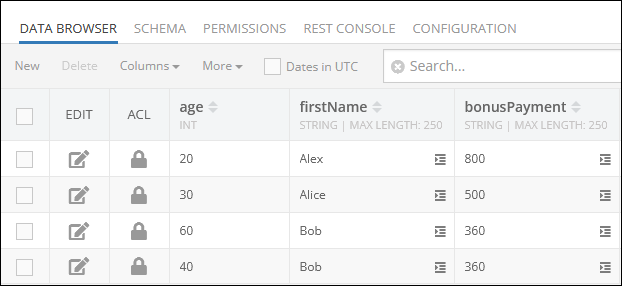This table contains the column `firstName`, and by passing the `true` value to the `setDistinct` method, only unique values will be returned in the response:

``````const query = Backendless.DataQueryBuilder.create()
query.setDistinct(true)

const result = await Backendless.Data.of('Employees').find(query)
``````

The query returns the following result which contains only unique names:

``````[
{
"___class": "Employees",
"firstName": "Alex"
},
{
"___class": "Employees",
"firstName": "Alice"
},
{
"___class": "Employees",
"firstName": "Bob"
}
]
``````

### Aggregate Functions¶

The `distinct` parameter can be also used with such aggregate functions as:

All examples presented further use sample data from the `Employees` data table presented in the beginning of this topic.

#### Average¶

Suppose you need to calculate an average value for a set of objects, but you want to skip identical records. The following example will retrieve only unique values from the `age` column and calculate the average age of all employees.

``````const query = Backendless.DataQueryBuilder.create()
query.setDistinct(true)

const result = await Backendless.Data.of('Employees').find(query)
``````

#### Count¶

The example below will count only unique values in the `firstName` column and return an integer value in the response:

``````const query = Backendless.DataQueryBuilder.create()
query.setDistinct(true)

const result = await Backendless.Data.of('Employees').find(query)
``````

#### Sum¶

The example below will calculate the sum of unique values in the `bonusPayment` column and return an integer value in the response:

``````const query = Backendless.DataQueryBuilder.create()
query.setDistinct(true)

const result = await Backendless.Data.of('Employees').find(query)
``````

### Codeless Reference¶

Consider the following data table called `Employees`: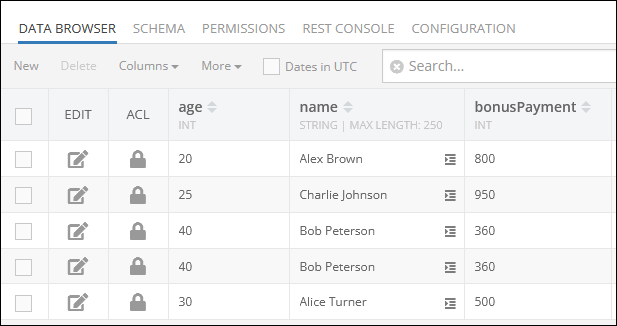#### AVERAGE¶

Suppose you need to calculate the average age of your employees, while excluding duplicates. As shown in the data table schema above, there are two identical records with the name `"Bob Peterson"`. that have the value of `40` in the `"age"` column.

The example below returns the average age only for unique records stored in the `Employees` data table: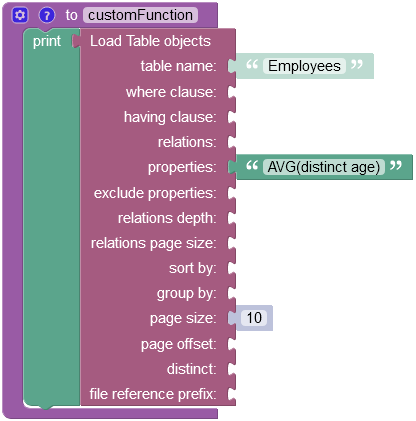The operation returns the following result in the response: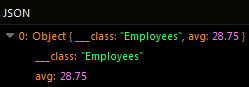#### COUNT¶

Suppose you need to count the number of employees, while excluding duplicates. Considering the data table schema provided above, there are two identical entities with the name `"Bob Peterson"`.

The example below counts the total number of unique employee `names` in the `Employees` data table: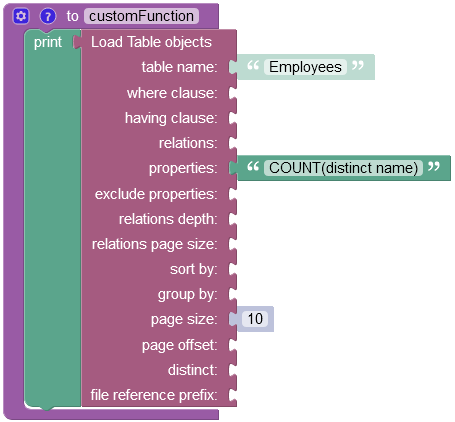The operation returns the following result in the response: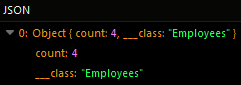#### SUM¶

Suppose you need to calculate the sum of all bonus payments, while excluding duplicates. Considering the data table schema provided above, there are two identical records that have the `360` value stored in the `"bonusPayment"` column:

The example below excludes the duplicate entity and calculates the sum of unique values: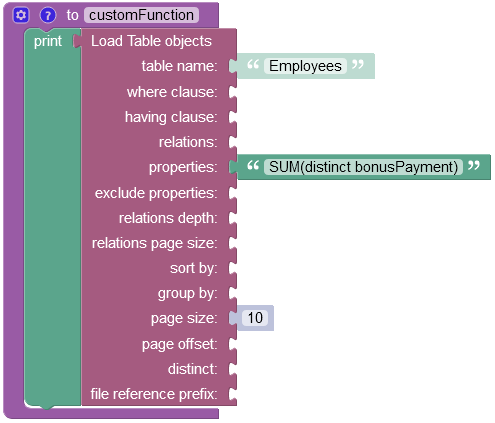The operation returns the following result in the response: